/

### Estimating operational risk capital requirements assuming data follows a gamma distribution (using the method of moments)

Suppose a firm has collated past operational annual loss data and the distribution of these annual losses is expected to provide a good guide to the distribution of future annual losses. Suppose the annual losses are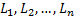. Suppose also that the losses come from a gamma distribution with probability density function: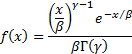where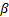is a scale parameter (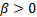) and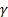is a shape parameter (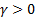).

One way of estimatingandis to use the ‘method of moments’ which for the gamma distribution can be done analytically.

We note that the gamma function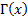is defined as: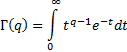This means that it has the property that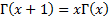for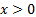. It also means (forand) that the following is true (which is necessary for the probability density of the gamma distribution to integrate to unity):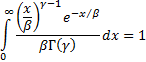We next note that the gamma distribution has the property that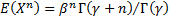. This is because: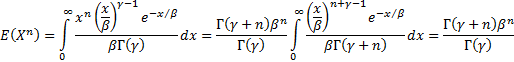To use the method of moments we will calculate the first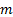moments of the observed data whereis the number of parameters to estimate. The gamma distribution has two parameters so here we have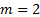and in this instance we calculate the first two moments (or equivalently the mean and variance) of the observed data and also the same two moments if the data comes from a gamma distribution with parametersandrespectively. We then select values ofandthat simultaneously equate the observed moments with the distributional moments.

For the gamma distribution we have: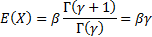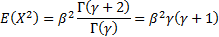So the mean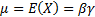and the variance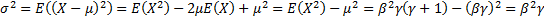.

The observed (sample) mean and variance [note it is not absolutely clear with method of moments whether to use the sample or population variance] are: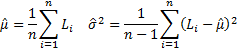So, with the method of moments we would selectandso that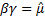,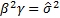, i.e.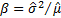and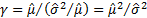.

If more recent observations were believed to be more relevant than less recent observations then we could give greater weight to more recent observations in the calculation of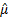and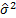, e.g. if the weights were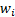then we might use (if we were focusing on population moments):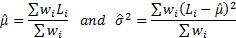[N.B. See here for insights on how to calculate weighted sample moments]

Another common method of estimating parameter values is to use maximum likelihood estimation, which cannot in general be done analytically for the gamma distribution but can be done numerically using e.g. the Nematrian website function MnProbDistMLE.

Of course, to estimate, say, a Value-at-Risk for operational risk you would ideally not just rely on past annual operational loss data. Additional information you might seek (and why you might seek it) includes:

From within the firm

Ideally, it would be helpful to have individual losses (so that you have amounts and frequency) allowing you to analyse loss size, i.e. severity, and loss frequency separately. Also desirable would be losses subdivided into appropriate categories, as this should provide extra colour on the losses being suffered. It would ideally also be helpful to have ‘volume’ statistics that are believed to be correlated with loss frequency. For example, operational losses might be expected to be linked to number of customers, staff turnover, funds under management etc. This sort of volume data should help you to estimate future annual losses better and also to get a better understanding of past behaviour.

From elsewhere

Hopefully the firm’s historic operational losses have been relatively small and have also been infrequent. Its own historical data may not therefore provide a good guide to the magnitude of large losses it might incur in the future. Access to other firms’ experience, including e.g. data collected by industry bodies, is therefore likely to be helpful, as long as the industry-wide experience is expected to be relevant to the firm.